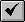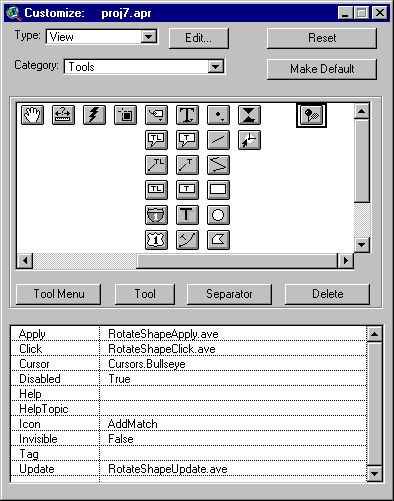English

# How To: Rotate features in a shapefile theme around a user selected point

## Summary

This article describes how to add to the View GUI a tool that rotates features within a shapefile.

## Procedure

1. Open three new script windows.

A. Activate the Project window.
B. Click the Scripts icon.
C. Click New.

2. Rename the scripts as follows.

A. Select Properties from the Script menu.
B. Type in a new name in the Name field.
C. Click OK.

RotateShapeApply.ave
RotateShapeClick.ave
RotateShapeUpdate.ave
3. Paste each of the following scripts into the corresponding script window.

`Code:'-- Script: RotateShapeApply.ave'-- Determine the selected featurestheView = av.GetActiveDoctheTheme = theView.GetEditableThemetheFTab = theTheme.GetFTabfldShape = theFTab.FindField("Shape")theSel = theFTab.GetSelection'-- Set the rotation anglerotAngle = System.GetEnvVar("Angle").AsNumberif (System.IsShiftKeyDown.Not) then  rotAngle = -1 * rotAngleend'-- Get the centre of rotation from the mouse clickrotPt = theView.GetDisplay.ReturnUserPointxs = rotPt.GetXys = rotPt.GetY'-- If the theme is a polyline or polygon theme...'-- Rotate the selected shapesif ((fldShape.GetType = #FIELD_SHAPELINE) or    (fldShape.GetType = #FIELD_SHAPEPOLY))  then   if (theFTab.StartEditingWithRecovery) then  for each r in theSel    shpIn = theFTab.ReturnValue(fldShape,r)    lstPoints = shpIn.AsList.Get(0)    newPoints = List.Make    for each pt in lstPoints      x1 = pt.GetX - xs      y1 = pt.Gety - ys      a1 = (y1 / x1).Atan      c = y1 / (a1.Sin)      a2 = (rotAngle * 0.01745329) + a1      x2 = a2.Cos * c      y2 = a2.Sin * c      xf = xs + x2      yf = ys + y2      newPt = Point.Make(xf,yf)      newPoints.Add(newPt)    end    if (shpIn.Is(Polygon)) then       shpOut = Polygon.Make({newPoints})    elseif (shpIn.Is(Polyline)) then       shpOut = Polyline.Make({newPoints})    else      exit    end        shpOut.Clean    theFTab.SetValue(fldShape,r,shpOut)    theFTab.EndTransaction  end  theView.SetSelectMode(#GRAPHICS_SELECT_NORMAL)  theTheme.Invalidate(True) endendif (fldShape.GetType = #FIELD_SHAPEPOINT) then   for each r in theSel    shpIn = theFTab.ReturnValue(fldShape,r)    pt = shpIn      x1 = pt.GetX - xs      y1 = pt.Gety - ys      a1 = (y1 / x1).Atan      c = y1 / (a1.Sin)      a2 = (rotAngle * 0.01745329) + a1      x2 = a2.Cos * c      y2 = a2.Sin * c      xf = xs + x2      yf = ys + y2      newPt = Point.Make(xf,yf)      shpOut = newPt    theFTab.SetValue(fldShape,r,shpOut)    theFTab.EndTransaction   end     theView.SetSelectMode(#GRAPHICS_SELECT_NORMAL)  theTheme.Invalidate(True)end'-- End of script: RotateShapeApply.ave `

`Code:'-- Script: RotateShapeClick.averotAngle = System.GetEnvVar("Angle")if (rotAngle = nil) then  rotAngle = "0"end  response = msgBox.Input("Enter rotation angle", "Rotate Shape",rotAngle)if (response = nil) then  exitelse  System.SetEnvVar("Angle",response)end'-- End of Script: RotateShapeClick.ave`

`Code:'-- Script: RotateShapeUpdate.avetheTheme = av.GetActiveDoc.GetEditableThemeif (theTheme = nil) then  selCount = 0else  selCount = theTheme.GetFTab.GetSelection.CountendSELF.SetEnabled(selCount > 0)'-- End of script: RotateShapeUpdate.ave `

4. Make each script active and select Compile from the Script menu or click thebutton.
5. Switch to the Project window and select Customize from the Project menu.
6. Select View from the Type dropdown list and Tools from the Category dropdown list.
7. Scroll to the far right of the Model tool bar and click the last tool.
8. Click the Separator button and then the Tool button.
9. Make the following settings:

`    Apply: RotateShapeApply.ave     Click: RotateShapeClick.ave    Cursor: Cursors.Bullseye  Disabled: true      Icon: Switch Select Invisible: False    Update: RotateShapeUpdate.ave`10. Close the Customize dialog box and open the view.
11. Make the shapefile theme active and select Start Editing from the Theme menu.
12. Select the features you wish to rotate.
13. Click the new tool and then click a point on the view.

`Note:The script uses this point as the axis of rotation.`

14. Type in the angle to rotate the selected features and click OK.